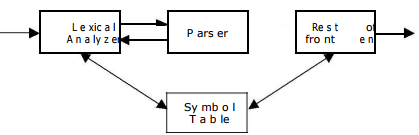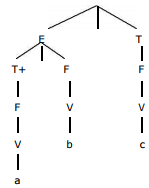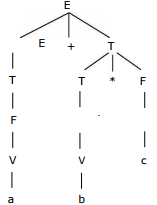Courses

# Context-Free Grammars: Definition & Parsing Notes | EduRev

## Computer Science Engineering (CSE) : Context-Free Grammars: Definition & Parsing Notes | EduRev

The document Context-Free Grammars: Definition & Parsing Notes | EduRev is a part of the Computer Science Engineering (CSE) Course Compiler Design.
All you need of Computer Science Engineering (CSE) at this link: Computer Science Engineering (CSE)

1. Context-free Grammars:
Definition: Formally, a context-free grammar G is a 4-tuple G = (V, T, P, S), where:
1. V is a finite set of variables (or nonterminals). These describe sets of “related” strings.
2. T is a finite set of terminals (i.e., tokens).
3. P is a finite set of productions, each of the form
A   where A  V is a variable, and   (V  T)* is a sequence of terminals and nonterminals. S  V is the start symbol.

Example of CFG: E ==>EAE | (E) | -E | id A==> + | - | * | / |

Where E, A are the non-terminals while id, +, *, -, /,(, ) are the terminals. 2. Syntax analysis:

In syntax analysis phase the source program is analyzed to check whether if conforms to the source language’s syntax, and to determine its phase structure. This phase is often separated into two phases:

• Lexical analysis: which produces a stream of tokens?
• Parser: which determines the phrase structure of the program based on the context-free grammar for the language?

2.1 PARSING: Parsing is the activity of checking whether a string of symbols is in the language of some grammar, where this string is usually the stream of tokens produced by the lexical analyzer. If the string is in the grammar, we want a parse tree, and if it is not, we hope for some kind of error message explaining why not.

There are two main kinds of parsers in use, named for the way they build the parse trees:

• Top-down: A top-down parser attempts to construct a tree from the root, applying productions forward to expand non-terminals into strings of symbols.
• Bottom-up: A Bottom-up parser builds the tree starting with the leaves, using productions in reverse to identify strings of symbols that can be grouped together.
In both cases the construction of derivation is directed by scanning the input sequence from left to right, one symbol at a time.

Parse Tree:A parse tree is the graphical representation of the structure of a sentence according to its grammar.

Example:
Let the production P is:
E T | E+T
T F | T*F
F V | (E)
V a | b | c |d

The parse tree may be viewed as a representation for a derivation that filters out the choice regarding the order of replacement.

Parse tree for a * b + cParse tree for a + b * c is:Parse tree for (a * b) * (c + d)Offer running on EduRev: Apply code STAYHOME200 to get INR 200 off on our premium plan EduRev Infinity!

## Compiler Design

16 videos|44 docs|29 tests

,

,

,

,

,

,

,

,

,

,

,

,

,

,

,

,

,

,

,

,

,

;